# Introduction

HTML tables can be scraped in a few lines using pandas' pd.read_html (), but this time I would like to show you how to scrape without using read_html ().

# Preparation

Install Beautiful Soup. (This time, we will also use pandas to create a data frame, so install it as appropriate.)

``````\$ pip install beautifulsoup4　# or conda install
``````

# policy

This time, as an example, let's get the following list of CPUs from this wikipedia page.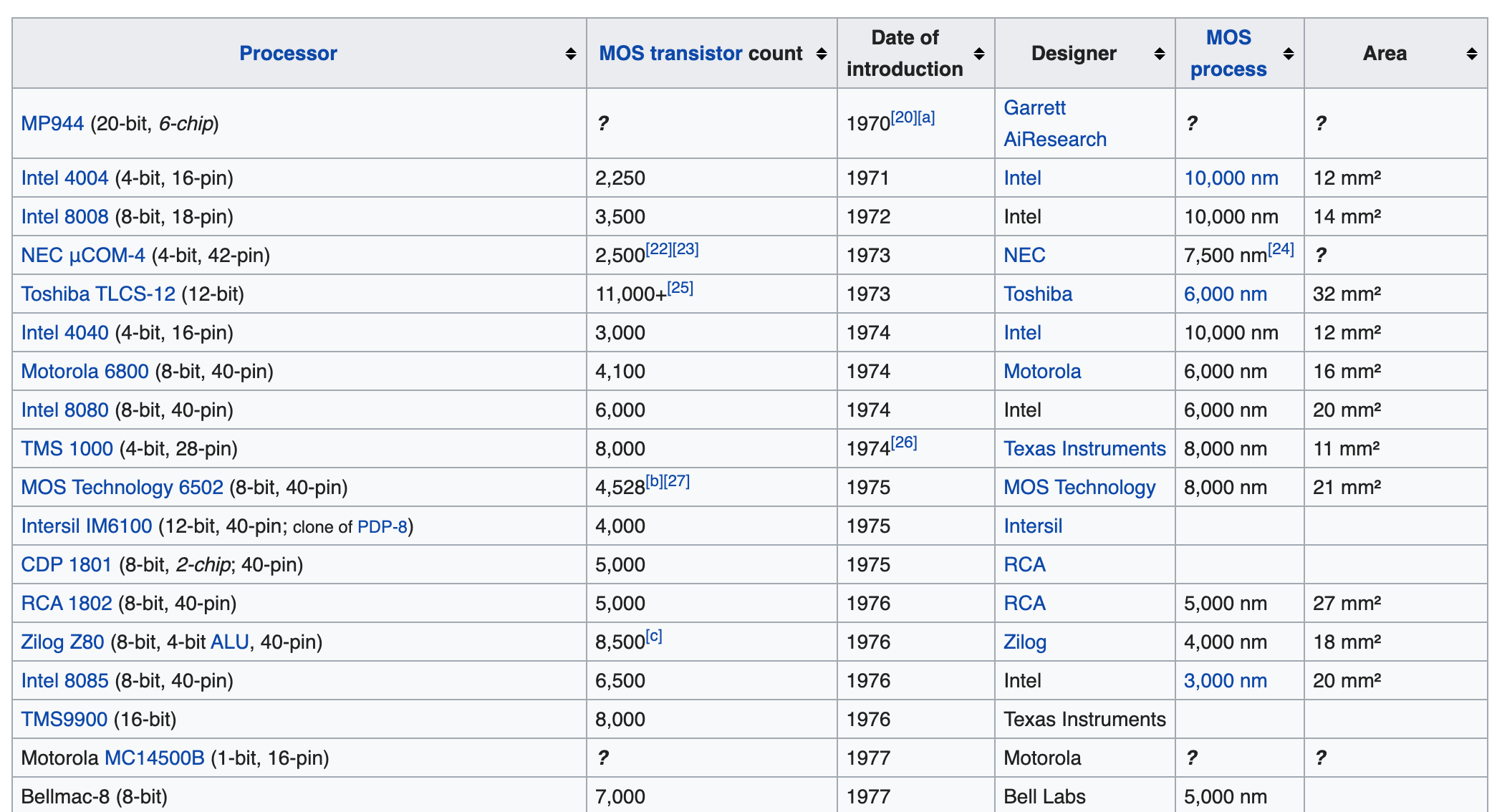#### reference

Here, for reference, I would like to show the method when using the super-easy method pd.read_html ().

``````import pandas as pd

url = 'https://en.wikipedia.org/wiki/Transistor_count'　#Target web page url
dfs = pd.read_html(url)　#If the web page has multiple tables, they will be stored in dfs in list format
``````

This time, it seems that the target table is stored in the first index of dfs, so let's output dfs (dfs  stores a table of another class).

``````dfs
``````

The output result looks like the image below, and you can certainly scrape it.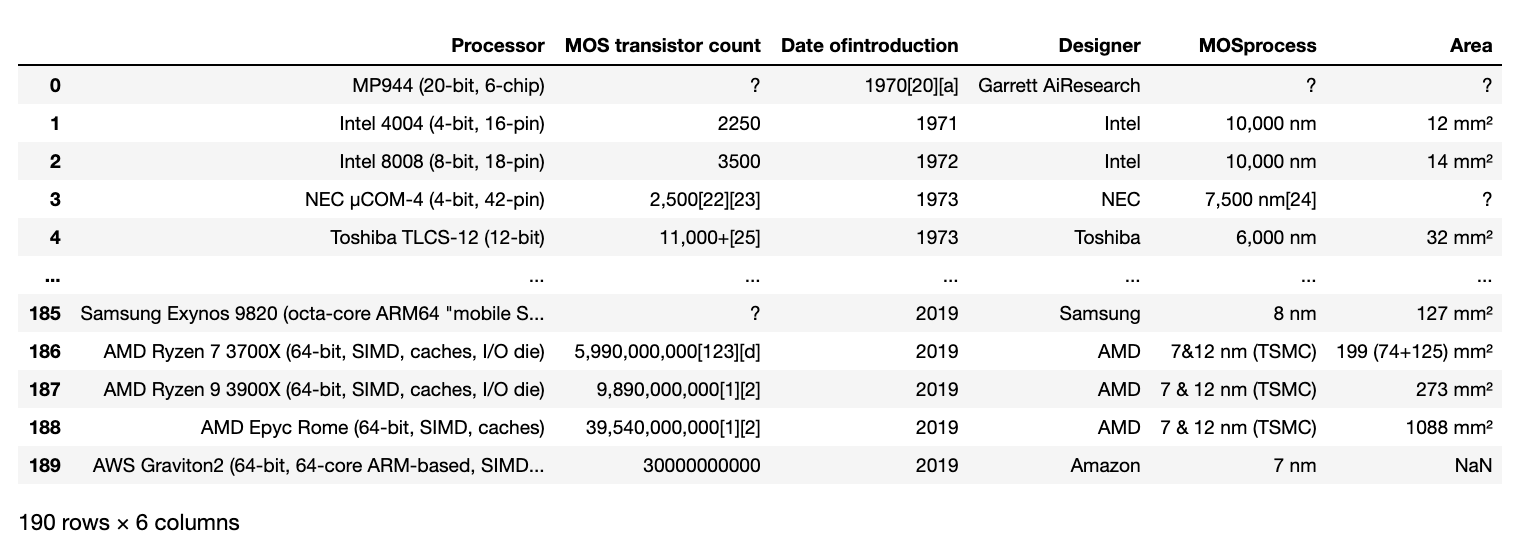# Overview

Before scraping a table with BeautifulSoup, let's take a look at the web page to which it is scraped. Let's jump to the wikipedia page from the link shown earlier and open the developer tools (in the case of chrome, you can display it by right-clicking on the table ⇒ inspect. You can also select option + command + I). Looking at the html source of the page with the developer tools, the target table is under the \

tag, \ (table body) ⇒ \ (table column component) ⇒ \ at the same level as the \ tag. , Corresponds to the column name (Processor ~ MOS process) part of the table).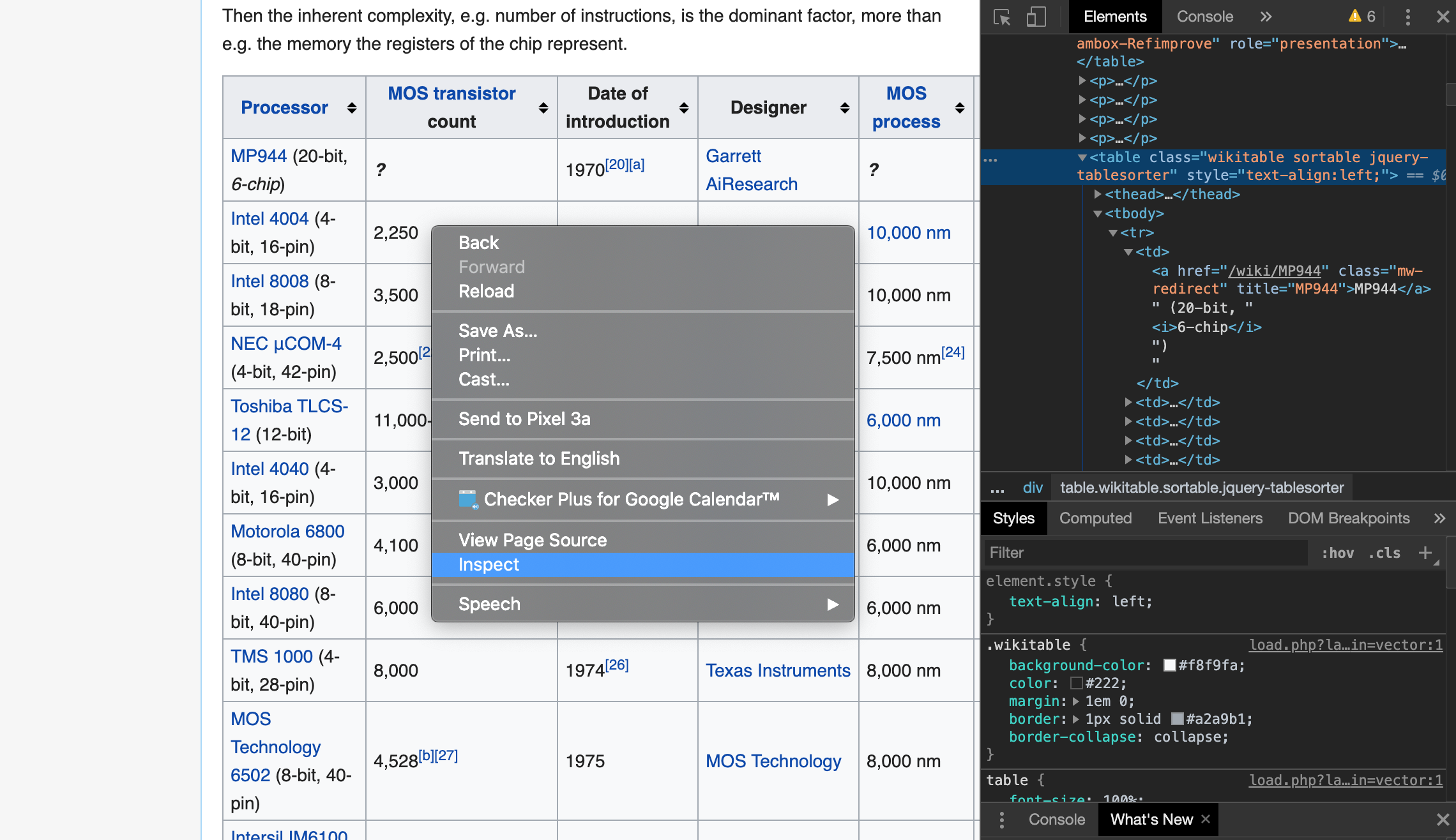# code

Let's write the code with the above overview in mind.

``````import requests
from bs4 import BeautifulSoup
import csv
import pandas as pd

url = "https://en.wikipedia.org/wiki/Transistor_count"
#Get web page data
page = requests.get(url)
#Parse html
soup = BeautifulSoup(page.text, 'html.parser')
``````

Let's take a look at the parsed data.

``````print(soup.prettify())
``````

As shown below, you can see the hierarchical structure as seen in the developer tools endlessly.

#### `output`

``````
<!DOCTYPE html>
<html class="client-nojs" dir="ltr" lang="en">
<meta charset="utf-8"/>
<title>
Transistor count - Wikipedia
</title>
<script>

・ ・ ・
``````

Let's extract the part that corresponds to the table. Use the find () method to extract the relevant part of the

tag by specifying the
 tag in the hierarchy below \ (table cell data) You can see that it has a hierarchical structure (not visible in the image below, but there is a \
tag and wikitable class.

``````table = soup.find('table', {'class':'wikitable'}).tbody
``````

You might think that you don't necessarily need to specify the table class, but you should specify it if there are tables of other classes. This time, as shown in the image below, it exists with another class name called box-More, so the wikitable class is explicitly specified.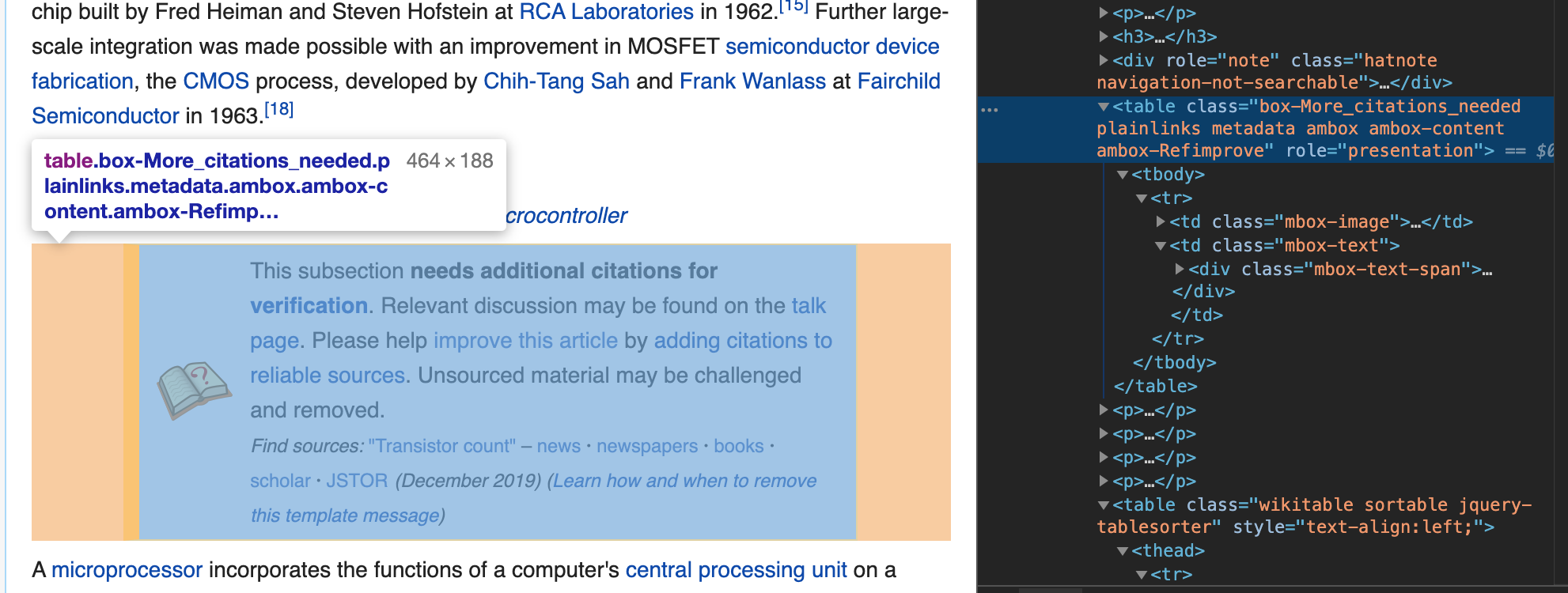Then, in the extracted table body, get the

tag part (row component of the table). The following find_all ('tr') stores each row component in list format.

``````
rows = table.find_all('tr')
``````

Let's look at the 0th element of the retrieved row component.

``````
print(rows)
``````

As shown below, there is an additional hierarchy of \

tag, and you can see that these correspond to the header part of the table.

#### `output`

``````

<tr>
<th><a href="/wiki/Microprocessor" title="Microprocessor">Processor</a>
</th>
<th data-sort-type="number"><a class="mw-redirect" href="/wiki/MOS_transistor" title="MOS transistor">MOS transistor</a> count
</th>
<th>Date of<br/>introduction
</th>
<th>Designer
</th>
<th data-sort-type="number"><a href="/wiki/MOSFET" title="MOSFET">MOS</a><br/><a href="/wiki/Semiconductor_device_fabrication" title="Semiconductor device fabrication">process</a>
</th>
<th data-sort-type="number">Area
</th></tr>
``````

On the other hand, let's look at the 0th next element of the acquired row components.

``````
print(rows)
``````

As you can see, there is an additional \

tag, which corresponds to the data component of each cell in the first row of the table.

#### `output`

``````

<tr>
<td><a class="mw-redirect" href="/wiki/MP944" title="MP944">MP944</a> (20-bit, <i>6-chip</i>)
</td>
<td><i><b>?</b></i>
</td>
<td>1970<sup class="reference" id="cite_ref-F-14_20-1"><a href="#cite_note-F-14-20"></a></sup><sup class="reference" id="cite_ref-22"><a href="#cite_note-22">[a]</a></sup>
</td>
<td><a href="/wiki/Garrett_AiResearch" title="Garrett AiResearch">Garrett AiResearch</a>
</td>
<td><i><b>?</b></i>
</td>
<td><i><b>?</b></i>
</td></tr>
``````

# Creating a data frame

Next, create a data frame from the extracted data. Let's start with the column name of the data frame. Gets all the \

tags inside the \
tag hierarchy inside the \
tags that are the header components from the 0th row of the table, and extracts only the text component (v.text).

``````
columns = [v.text for v in rows.find_all('th')]
print(columns)
``````

The result is as follows, but \ n indicating a line break is an obstacle.

#### `output`

``````
['Processor\n', 'MOS transistor count\n', 'Date ofintroduction\n', 'Designer\n', 'MOSprocess\n', 'Area\n']
``````

So let's modify the above code as follows.

``````
columns = [v.text.replace('\n', '') for v in rows.find_all('th')]
print(columns)
``````

The result is as follows. Only the column name could be extracted neatly.

#### `output`

``````
['Processor', 'MOS transistor count', 'Date ofintroduction', 'Designer', 'MOSprocess', 'Area']
``````

Now, let's prepare an empty data frame by specifying the above column name.

``````
df = pd.DataFrame(columns=columns)
df
``````

The result is as follows. Only the column name is displayed in the header part, and you can see the empty data frame.Now that we have extracted the columns, let's extract each data component of the table.

``````#About a certain row component of all rows
for i in range(len(rows)):
#All of<td>Get tags (cell data), store them in tds, and list them
tds = rows[i].find_all('td')
#Exclude cases where the number of tds data does not match the number of columns (blank), etc.
if len(tds) == len(columns):
#Store and list all cell data (of a certain row component) as text components in values
values = [ td.text.replace('\n', '').replace('\xa0', ' ') for td in tds ]
#values pd.Convert to series data, combine to data frame
df = df.append(pd.Series(values, index=columns), ignore_index= True)
``````

Let's output the created data frame.

``````df
``````

The result should look like the image below. I was able to scrape the table cleanly with Beautiful Soup.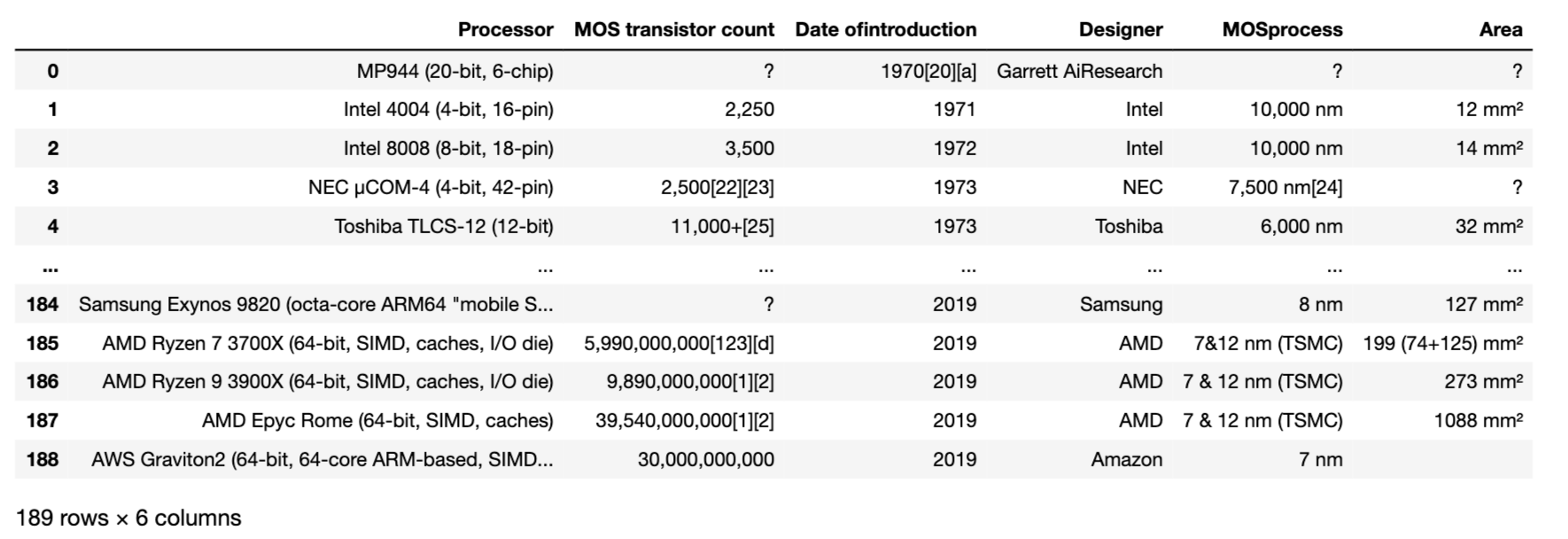By the way, if the above td.text.replace ('\ n',''). replace ('\ xa0','') is simply executed as td.text, the values will be as follows. (A component of values is shown as an example).

#### `output`

``````
['Intel 4004 (4-bit, 16-pin)\n', '2,250\n', '1971\n', 'Intel\n', '10,000\xa0nm\n', '12\xa0mm²\n']
``````

As with the header, the line feed code \ n and the space code \ xa0 are included. Therefore, it is necessary to replace each with the replace () method.

Save the created data frame in csv format as appropriate.

``````#No header, tab specified for delimiter
df.to_csv('processor.csv', index=False, sep='\t' )
``````

# Code summary

``````import requests
from bs4 import BeautifulSoup
import csv
import pandas as pd

url = 'https://en.wikipedia.org/wiki/Transistor_count'
page = requests.get(url)

soup = BeautifulSoup(page.text, 'html.parser')
table = soup.find('table', {'class':'wikitable'}).tbody

rows = table.find_all('tr')
columns = [v.text.replace('\n', '') for v in rows.find_all('th')]

df = pd.DataFrame(columns=columns)

for i in range(len(rows)):
tds = rows[i].find_all('td')

if len(tds) == len(columns):
values = [ td.text.replace('\n', '').replace('\xa0', ' ') for td in tds ]
df = df.append(pd.Series(values, index=columns), ignore_index= True)

df.to_csv('processor.csv', index=False, sep='\t' )
``````